# Relative Motion in Two Dimensions

Now we turn from relative motion in one dimension to relative motion in two (and, by extension, in three) dimensions. In Fig. 4-21, our two observers are again watching a moving particle P from the origins of reference frames A and B, while В moves at a constant velocity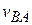relative to A. (The corresponding axes of these two frames remain parallel.)

Figure 4-21 shows a certain instant during the motion. At that instant, the position vector of В relative to A is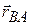. Also, the position vectors of particle P are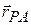relative to A and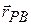relative to B. From the arrangement of heads and tails of those three position vectors, we can relate the vectors with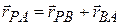(4-41)The plane has velocity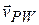relative to the wind, with an airspeed (speed relative to the wind) of 215 km/h, directed at angle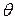south of east. The wind has velocity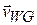relative to the ground, with a speed of 65.0 km/h, directed 20.0° east of north. What is the magnitude of the velocity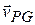of the plane relative to the ground, and what is 0?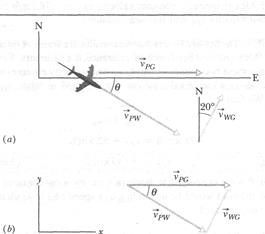Fig. 4-21 Frame В has the constant two-dimensional velocity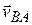relative to frame A. The position vector of В relative to A is. The position vectors of particle P arerelative to A andrelative to B.

By taking the time derivative of this equation, of particle P we can relate the velocities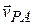and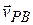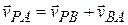By taking the time derivative of this relation, we can relate the accelerations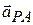and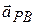of the particle P relative to our observers. However, note that becauseis constant, its time derivative is zero. Thus, we get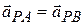As for one-dimensional motion, we have the following rule: Observers on different frames of reference that move at constant velocity relative to each other will measure the same acceleration for a moving particle.Sample Problem 4-11

In Fig. 4-22a, a plane moves due east (directly toward the east) while the pilot points the plane somewhat south of east, toward a steady wind that blows to the northeast. SOLUTION: The Key Idea is that the situation is like the one in Fig. 4-21. Here the moving particle P is the plane, frame A is attached to the ground (call it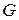), and frame В is "attached" to the wind (call it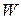). We need to construct a vector diagram like that in Fig. 4-21 but this time using the three velocity vectors.

First construct a sentence that relates the three vectors: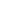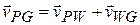We want the magnitude of the first vector and the direction of the second vector. With unknowns in two vectors, we cannot solve Eq. 4-44 directly on a vector-capable calculator. Instead, we need to resolve the vectors into components on the coordinate system of Fig. 4-226, and then solve Eq. 4-44 axis by axis (see Section 3-5). For the у components, we find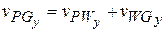or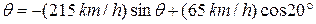Solving forgives us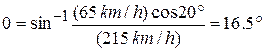(Answer)

Similarly, for the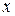components we find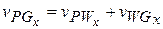Here, becauseis parallel to theaxis, the component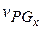is equal to the magnitude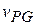. Substituting this and=16.5°, we find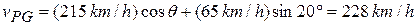(Answer)

A body moves in a straight line along-axis. Its distances(in meter) from the origin is given by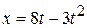. The average speed in the interval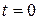to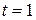second is

(A) 5 m/s (B) -4 m/s

(C) 6 m/s (D) zero

A particle moves along-axis in such a way that its coordinatevanes with time t according to the expression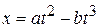. The acceleration of the particle will be zero at time

(A)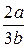(B)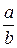(C)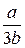(D) zero

A particle moves along a straight line, such that its displacement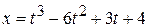(in metres). The velocity, when the acceleration is zero, is

(A) -12 m/s (B) -9 m/s (C) 3 m/s (D) 42 m/s

The equation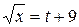gives the variation of displacement with time. Which of the following is correct?

(A) Velocity is proportional to time.

(B) Velocity is inversely proportional to time.

(C) Acceleration depends upon time.

(D) Acceleration is constant.

A particle moving along a straight line has a velocity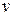m/s, when it cleared a distance of x meters. These two are connected by the relation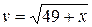. When its velocity is l m/s, its acceleration (in m s-2) is :

(A) 2 (B) 7 (C) 1 (D) 0.5

If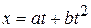, where x is the distance traveled by the body kilometers, while t is time in seconds, then units of b

(A) km/s (B) km·s (C) km/s2 (D) km·s2

An acceleration of a particle is increasing linearly with time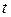as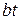The particle starts from the origin with an initial velocity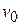. The distance traveled by the particle in timewill be

(A)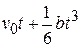(B)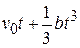(C)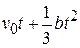(D)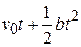Дата добавления: 2015-06-17; просмотров: 753;

Поиск по сайту:

При помощи поиска вы сможете найти нужную вам информацию.

Поделитесь с друзьями:

Если вам перенёс пользу информационный материал, или помог в учебе – поделитесь этим сайтом с друзьями и знакомыми.
helpiks.org - Хелпикс.Орг - 2014-2023 год. Материал сайта представляется для ознакомительного и учебного использования. | Поддержка
Генерация страницы за: 0.014 сек.